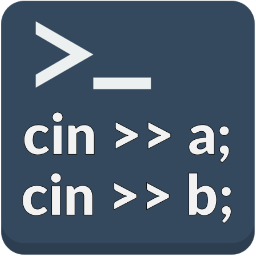#Nakov.IO.Cin 2.0.2.1

.NET Standard 2.0 .NET Framework 2.0
`dotnet add package Nakov.IO.Cin --version 2.0.2.1`
`NuGet\Install-Package Nakov.IO.Cin -Version 2.0.2.1`
This command is intended to be used within the Package Manager Console in Visual Studio, as it uses the NuGet module's version of Install-Package.
`<PackageReference Include="Nakov.IO.Cin" Version="2.0.2.1" />`
For projects that support PackageReference, copy this XML node into the project file to reference the package.
`paket add Nakov.IO.Cin --version 2.0.2.1`
`#r "nuget: Nakov.IO.Cin, 2.0.2.1"`
#r directive can be used in F# Interactive and Polyglot Notebooks. Copy this into the interactive tool or source code of the script to reference the package.
```// Install Nakov.IO.Cin as a Cake Addin

// Install Nakov.IO.Cin as a Cake Tool
#tool nuget:?package=Nakov.IO.Cin&version=2.0.2.1```

## C++ style `cin` console-based input for C# developers

`Nakov.IO.Cin` is a console-based input parser for C#, which reads numbers and text in the C++ `cin` / `cout` / `iostream` style.

For example, in C++ we can read two integers using this code:

``````int x, y;
cin >> x >> y;
``````

With `Nakov.IO.Cin` we can write the same code in C# like this:

``````int x = Cin.NextInt();
int y = Cin.NextInt();
``````

Like in C++, the whitespace will be skipped so users can enter the input numbers on the same line, separated by spaces, or on separate lines.

`Nakov.IO.Cin` supports reading `int`, `double`, `decimal` and `string` tokens.

### Examples

In this example we read two integers in C++:

``````int rows, cols;
cin >> rows >> cols;
``````

In C# we can read two integers (using tuples) like this:

``````(int rows, int cols) = (Cin.NextInt(), Cin.NextInt());
``````

Another C++ example:

``````#include <iostream>

using namespace std;

int main()
{
int n;
cin >> n;

int* numbers = new int[n];
for (int i = 0; i < n; i++)
cin >> numbers[i];

for (int i = 0; i < n; i++)
cout << numbers[i] << ' ';
}
``````

The corresponding C# code:

``````using System;
using Nakov.IO;

public class EnterNumbers
{
static void Main()
{
int n = Cin.NextInt();

int[] numbers = new int[n];
for (int i = 0; i < n; i++)
numbers[i] = Cin.NextInt();

for (int i = 0; i < n; i++)
Console.Write(numbers[i] + " ");
}
}
``````

### More Detailed Example

This is a more-detailed example how to use the `Nakov.IO.Cin` class:

``````using System;
using Nakov.IO; // See http://www.nakov.com/tags/cin

public class CinExample
{
static void Main()
{

Console.Write("Enter two integers x and y separated by whitespace: ");
// cin >> x >> y;
int x = Cin.NextInt();
double y = Cin.NextDouble();

Console.WriteLine("Name: {0}, Age: {1}", name, age);
Console.WriteLine("x={0}, y={1}", x, y);

Console.Write("Enter a positive integer number N: ");
// cin >> n;
int n = Cin.NextInt();

Console.Write("Enter N decimal numbers separated by a space: ");
decimal[] numbers = new decimal[n];
for (int i = 0; i < n; i++)
{
// cin >> numbers[i];
numbers[i] = Cin.NextDecimal();
}

Array.Sort(numbers);
Console.WriteLine("The numbers in ascending order: {0}",
string.Join(' ', numbers));

Console.Write("Enter two strings seperated by a space: ");
// cin >> firstStr >> secondStr;
string firstStr = Cin.NextToken();
string secondStr = Cin.NextToken();
Console.WriteLine("First str={0}", firstStr);
Console.WriteLine("Second str={0}", secondStr);
}
}
``````

This is a sample input and output from the above example:

``````Enter your name: Albert Einstein
Enter two integers x and y separated by whitespace:
10
20
Name: Albert Einstein, Age: 25
x=10, y=20
Enter a positive integer number N:
5
Enter N decimal numbers separated by a space: 10  30 40

50
20
The numbers in ascending order: 10 20 30 40 50
Enter two strings seperated by a space:
Visual                  Studio
First str=Visual
Second str=Studio
``````

Note that input numbers and string tokens can be separated by single space, by a new line or by a sequence of white space characters.

Product Compatible and additional computed target framework versions.
.NET net5.0 was computed.  net5.0-windows was computed.  net6.0 was computed.  net6.0-android was computed.  net6.0-ios was computed.  net6.0-maccatalyst was computed.  net6.0-macos was computed.  net6.0-tvos was computed.  net6.0-windows was computed.  net7.0 was computed.  net7.0-android was computed.  net7.0-ios was computed.  net7.0-maccatalyst was computed.  net7.0-macos was computed.  net7.0-tvos was computed.  net7.0-windows was computed.
.NET Core netcoreapp2.0 was computed.  netcoreapp2.1 was computed.  netcoreapp2.2 was computed.  netcoreapp3.0 was computed.  netcoreapp3.1 was computed.
.NET Standard netstandard2.0 is compatible.  netstandard2.1 was computed.
.NET Framework net20 is compatible.  net35 was computed.  net40 was computed.  net403 was computed.  net45 was computed.  net451 was computed.  net452 was computed.  net46 was computed.  net461 was computed.  net462 was computed.  net463 was computed.  net47 was computed.  net471 was computed.  net472 was computed.  net48 was computed.  net481 was computed.
MonoAndroid monoandroid was computed.
MonoMac monomac was computed.
MonoTouch monotouch was computed.
Tizen tizen40 was computed.  tizen60 was computed.
Xamarin.iOS xamarinios was computed.
Xamarin.Mac xamarinmac was computed.
Xamarin.TVOS xamarintvos was computed.
Xamarin.WatchOS xamarinwatchos was computed.
Compatible target framework(s)
• #### .NETFramework 2.0

• No dependencies.
• #### .NETStandard 2.0

• No dependencies.

### NuGet packages

This package is not used by any NuGet packages.

### GitHub repositories

This package is not used by any popular GitHub repositories.